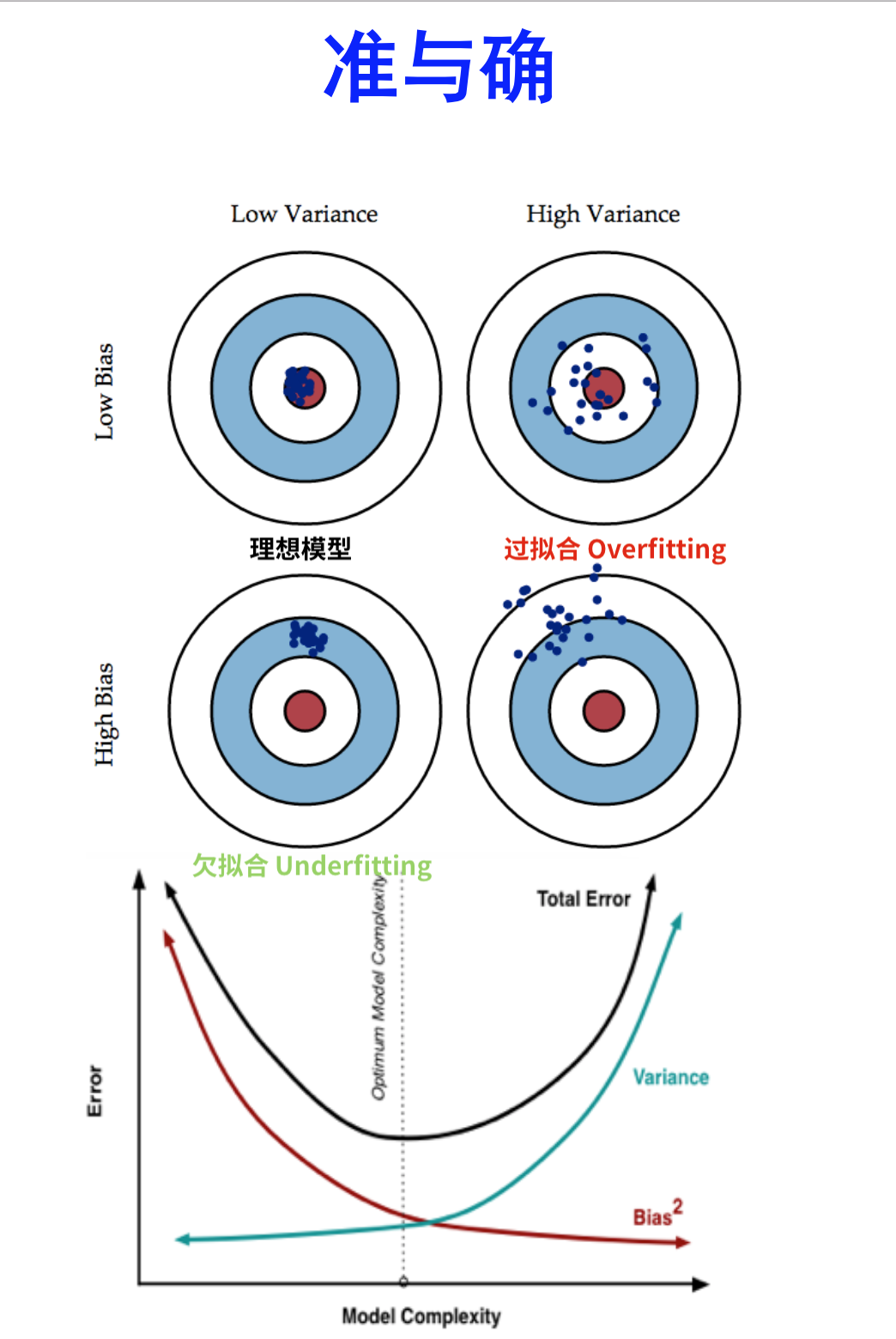【阅读时间】16 - 26 min
【内容简介】系统详解分类器性能指标，什么是准确率 - Accuracy精确率 - Precision召回率 - RecallF1值ROC曲线AUC曲线误差 - Error偏差 - Bias方差 - VarianceBias-Variance Tradeoff

# 混淆矩阵 - Confusion Matrix

• 一个实例是正类，并被预测为正类，记为真正类（True Positive TP/T+
• 一个实例是正类，但被预测为负类，记为假负类（False Negative FN/F-
• 一个实例是负类，但被预测为正类，记为假正类（False Positive FP/F+
• 一个实例是负类，但被预测为负类，记为真负类（True Negative TN/F-

TP和TN中的真表示分类正确，同理FN和FP表示分类错误的

TPR True Positive Rate 真正类率 Recall Sensitivity $\frac {\sum T+}{\sum C+}$
FNR False Negative Rate 假负类率Miss rate Type rs error $\frac {\sum F-}{\sum C+}$
FPR False Positive Rate 假正类率fall-out Type 1 error $\frac {\sum F+}{\sum C-}$
TNR Tre Negative Rate 真负类率Specificity $\frac {\sum T-}{\sum C-}$

PPV Positive Predictive Value 正类预测率Precision $\frac {\sum T+}{\sum Pc+}$
FOR False Omission Rata 假错误率 $\frac {\sum F-}{\sum Pc-}$
FDR False Discovery Rate 假发现率 $\frac {\sum F+}{\sum Pc+}$
NPV Negative Predictive Value 负类预测率 $\frac {\sum T-}{\sum Pc-}$

ACC Accuracy 准确率 $\frac {\sum (T+) + \sum {T-}}{样本空间}$
LR+ Positive Likelihood Ratio 正类似然比 $\frac {TPR}{FPR}$
LR- Negative likelihood ratio 负类似然比 $\frac {FNR}{TNR}$
DOR Diagnostic odds ratio 诊断胜算比 $\frac {LR+}{LR-}$
F1 score $F_1$ test measure F1值 $\frac{2}{\frac{1}{recall}+\frac{1}{precision}}$
MCC Matthews Correlation coefficient 马修斯相关性系数 $\frac{TP \times TN - FP \times FN}{\sqrt {(TP + FP)(TP + FN)(TN + FP)(TN +FN)}}$

LR+/-指的是似然比，LR+ 越大表示模型对正类的分类越好，LR-越大表示模型对负类的分类效果越好

F1值是精确值和召回率的调和均值，其实原公式是 $F_\beta = (1 + \beta^2)\frac{precision \times recall}{(\beta^2recall)+recall}$，这里的β表示：召回率的权重是准确率的β倍。即F值是一种精确率和召回率的综合指标，权重由β决定

MCC值在[-1,1]之间，靠近1表示完全预测正确，靠近-1表示完全悖论，0表示随机预测

# ROC Curve

ROC - Receiver Operating Characteristic Curve，接受者操作特征曲线，ROC曲线

## 什么是ROC曲线

ROC曲线和混淆矩阵息息相关，上一部分已经详细解释了相关内容，这里直接说明ROC曲线的横坐标和纵坐标分别是什么

• 第一个点：(0,1)，FPR=0 TPR=1 ，这意味着所有的正类全部分类正确，或者说这是一个完美的分类器，将所有的样本都分类正确了
• 第二个点：(1,0)， FPR=1 TPR=0 ，和第一个点比较，这是第一个点的完全反面，意味着是个最糟糕的分类器，将所有的样本都分类错误了（但其实可以直接取反，就是最好的模型，因为是二分类问题）
• 第三个点：(0,0)，FPR=0 TPR=0 也就是原点，这个点表示的意思是，分类器预测所有的样本都为负类
• 第四个点：(1,1)，FPR=1 TPR=1，和第三个点对应，表示分类器预测所有的样本都为正类
• 一条线：y=x。这条对角线上的点实际上就是一个采用随机猜测策略的分类器的结果

$TPR = \frac{TP}{TP+FN}$ $FPR = \frac{FP}{FP+TN}$ 蓝色图像是正类分类器的概率分布，红色图像负类分类器的概率分布，竖直的黑线是阈值（Threshold），二分类分类器的输出就是一个取值在[0,1]间的值（概率），我们将黑线从0移动到1，就能得出一条曲线，这条线就是ROC曲线

## 为什么使用ROC曲线

ROC曲线有一个很好的特性：当测试集中的正负样本的分布变化的时候，ROC曲线能够保持不变。在实际的数据集中经常会出现类不平衡（class imbalance）现象，即负样本比正样本多很多（或者相反），而且测试数据中的正负样本的分布也可能随着时间变化。下图是ROC曲线和Precision-Recall曲线的对比：

(a)和(b)展示的是分类其在原始测试集（正负样本分布平衡）的结果，(c)和(d)是将测试集中负样本的数量增加到原来的10倍后，分类器的结果。可以明显的看出，ROC曲线基本保持原貌，而Precision-Recall曲线则变化较大，记住这个结论即可

# AUC Value

AUC - Area Under Curve被定义为ROC曲线下的面积

AUC在[0.5,1]之间，这是因为ROC曲线一般都处于y=x这条直线的上方（否则这个做分类器的人连简单的取非都不会真可以去死了）

AUC值越大，证明这个模型越好

## 三个名词表示了什么• ：Bias 描述的是根据样本训练的模型的输出预测结果的期望样本真实结果的差距，说人话，这个模型对样本拟合的好不好。想在Bias上表现好，降低Bias，就是复杂化模型，增加模型的参数，但这样容易过拟合（Overfitting）Low Bias对应的就是点都在靶心附近，所以瞄的都是准的，但手不一定稳
• ：Variance 描述的是根据样本训练的模型在测试集上的表现（泛化能力） ，想在Variance上表现好，降低Variance，需要简化模型，减少模型的参数，这样做容易欠拟合，对应上图的High Bias，点偏离的中心。Low Variance对应的是点打的都很集中，但不一定在靶心附近，手很稳，但是瞄的不准

$$E[(y - \hat f(x))^2] = Bias[\hat f(x)]^2 + Var[\hat f(x)] + \sigma^2$$

Bias-Variance Tradeoff作为机器学习一个核心训练的观点或者说概念，推导觉得还是十分重要，整理如下

## 推导过程

$$Var[X] = E[X^2] - E[X]^2 \implies E[X^2] = Var[X] + E[X]^2$$

$$E[y] = E[f + \epsilon] = E[f] = f$$

$$Var[y] = E[(y-E[y])^2] = E[(y - f)^2] = E[(f + \epsilon - f)^2] = E[\epsilon^2] = Var[\epsilon] + E[\epsilon]^2 = \sigma^2$$ 由于 $\epsilon$ 和 $\hat f$ 互相独立 \begin{align} E[(y - \hat f)^2] & = E[y^2 + \hat f^2 - 2y\hat f] \\ & = E[y^2] + E[\hat f^2] - E[2y\hat f] \\ & = Var[y] + E[y]^2 + Var[\hat f] + E[\hat f]^2 - 2fE[\hat f] \\ & = Var[y] + Var[\hat f] + (f^2 - 2fE[\hat f] + E[\hat f]^2) \\ & = Var[y] + Var[\hat f] + (f - E[\hat f])^2 \\ & = \sigma^2 + Var[\hat f] + Bias[\hat f]^2 \end{align}